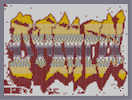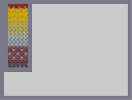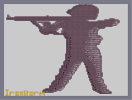### Damca!Hover over the thumbnail for a full-size version.

Author ironstorm author:ironstorm graffiti n-art nonplayable rated 2008-09-11 2008-09-11 4 by 13 people. \$Damca!#ironstorm#none#00000000000000000000000000000000000000000000000000000000000000000000000000000000000000000000000000000000000000000000000000000000000000000000000000000000000000000000000000000000000000000000000000000000000000000000000000000000000000000000000000000000000000000000000000000000000000000000000000000000000000000000000000000000000000000000000000000000000000000000000000000000000000000000000000000000000000000000000000000000000000000000000000000000000000000000000000000000000000000000000000000000000000000000000000000000000000000000000000000000000000000000000000000000000000000000000000000000000000000000000000000000000000000000000000000000000000000000000000000000000000000000000000000000000000000000000000000000000000000|12^24,24!12^24,30!12^24,36!12^30,36!12^30,30!12^30,24!12^36,30!12^42,36!12^36,36!12^66,36!12^24,54!12^30,54!12^42,132!12^186,48!12^120,72!12^114,78!12^126,78!12^108,84!12^108,90!12^102,96!12^96,102!12^90,108!12^84,114!12^78,114!12^72,120!12^66,126!12^66,132!12^66,138!12^66,144!12^66,150!12^66,162!12^66,156!12^66,168!12^72,168!12^78,168!12^84,168!12^84,174!12^84,180!12^84,186!12^60,174!12^60,180!12^60,186!12^60,198!12^66,198!12^66,192!12^66,174!12^72,174!12^78,174!12^78,186!12^72,186!12^66,186!12^66,180!12^72,180!12^78,180!12^72,198!12^78,198!12^78,192!12^72,192!12^72,204!12^72,210!12^72,216!12^78,204!12^66,216!12^72,222!12^72,228!12^72,234!12^72,240!12^72,246!12^72,252!12^72,258!12^66,240!12^78,246!12^78,252!12^84,252!12^60,252!12^54,258!12^54,264!12^48,270!12^36,258!12^36,264!12^36,276!12^42,282!12^42,288!12^48,288!12^54,294!12^60,300!12^66,306!12^72,312!12^78,318!12^84,318!12^84,312!12^78,312!12^78,306!12^78,300!12^84,300!12^84,294!12^84,306!12^36,294!12^42,294!12^48,294!12^48,300!12^48,306!12^48,312!12^42,318!12^42,324!12^30,324!12^48,318!12^54,300!12^54,306!12^54,312!12^48,324!12^54,324!12^60,324!12^60,318!12^60,312!12^66,306!12^54,318!12^66,306!12^66,306!12^60,306!12^66,312!12^66,318!12^66,324!12^72,324!12^78,324!12^72,318!12^72,318!12^54,330!12^54,336!12^48,342!12^60,336!12^66,336!12^72,336!12^78,336!12^78,330!12^72,330!12^66,330!12^60,330!12^54,348!12^60,342!12^66,342!12^72,342!12^78,342!12^60,348!12^66,348!12^72,348!12^78,348!12^66,354!12^72,354!12^78,354!12^72,360!12^78,360!12^78,366!12^72,366!12^66,366!12^60,366!12^54,372!12^54,378!12^30,390!12^30,396!12^54,402!12^54,408!12^48,408!12^48,414!12^54,414!12^60,414!12^60,420!12^54,420!12^48,420!12^60,426!12^66,426!12^66,432!12^60,432!12^60,438!12^66,438!12^72,438!12^42,432!12^42,438!12^54,444!12^60,444!12^66,444!12^72,444!12^48,450!12^54,450!12^60,450!12^66,450!12^72,450!12^78,450!12^90,450!12^96,456!12^90,456!12^84,456!12^78,456!12^72,456!12^66,456!12^54,456!12^48,456!12^42,462!12^60,462!12^66,462!12^72,462!12^78,462!12^84,462!12^90,462!12^96,462!12^60,468!12^72,468!12^78,468!12^84,468!12^90,468!12^96,468!12^102,468!12^108,468!12^114,468!12^120,468!12^126,468!12^132,468!12^138,468!12^144,468!12^156,468!12^150,468!12^162,468!12^108,462!12^114,462!12^120,462!12^126,462!12^132,462!12^138,462!12^144,462!12^174,474!12^168,474!12^162,474!12^156,474!12^150,474!12^144,474!12^132,474!12^138,474!12^126,474!12^120,474!12^114,474!12^108,474!12^102,474!12^96,474!12^90,474!12^84,474!12^78,474!12^72,474!12^66,474!12^66,480!12^66,486!12^54,486!12^72,492!12^180,480!12^78,480!12^84,480!12^90,480!12^96,480!12^102,480!12^108,480!12^114,480!12^120,480!12^126,480!12^132,480!12^138,480!12^144,480!12^150,480!12^156,480!12^162,480!12^168,480!12^174,480!12^186,480!12^186,474!12^192,468!12^198,468!12^78,486!12^84,486!12^90,486!12^96,486!12^102,486!12^108,486!12^114,486!12^120,486!12^126,486!12^132,486!12^138,486!12^144,486!12^150,486!12^156,486!12^162,486!12^168,486!12^174,486!12^180,486!12^192,480!12^192,474!12^198,474!12^192,462!12^192,456!12^198,480!12^204,468!12^204,486!12^198,486!12^186,486!12^210,468!12^210,474!12^210,480!12^210,486!12^186,492!12^186,498!12^186,504!12^186,504!12^186,510!12^204,492!12^204,498!12^204,504!12^204,510!12^210,510!12^210,504!12^216,504!12^228,504!12^228,510!12^222,504!12^222,510!12^210,492!12^216,492!12^222,498!12^228,492!12^234,504!12^240,504!12^246,504!12^246,492!12^240,492!12^234,492!12^252,492!12^252,498!12^252,504!12^84,492!12^84,498!12^78,504!12^90,504!12^96,504!12^102,504!12^114,504!12^108,504!12^90,498!12^96,498!12^102,498!12^108,498!12^114,498!12^90,492!12^96,492!12^102,492!12^108,492!12^114,492!12^120,492!12^126,492!12^132,492!12^138,492!12^144,492!12^150,492!12^156,492!12^162,492!12^168,492!12^174,492!12^180,492!12^180,498!12^102,510!12^102,516!12^108,516!12^114,516!12^120,516!12^126,516!12^132,516!12^138,516!12^180,516!12^174,516!12^168,516!12^162,516!12^156,516!12^150,516!12^144,516!12^108,510!12^114,510!12^120,510!12^120,504!12^120,498!12^126,498!12^132,498!12^138,498!12^138,498!12^144,498!12^150,498!12^156,498!12^162,498!12^168,498!12^168,498!12^174,498!12^174,504!12^180,504!12^180,510!12^174,510!12^168,510!12^162,510!12^156,510!12^150,510!12^144,510!12^138,510!12^132,510!12^126,510!12^126,504!12^132,504!12^138,504!12^144,504!12^150,504!12^156,504!12^162,504!12^168,504!12^180,522!12^180,528!12^174,534!12^174,528!12^168,528!12^162,528!12^156,522!12^150,522!12^162,522!12^168,522!12^174,522!12^186,534!12^192,534!12^192,540!12^186,540!12^186,546!12^180,546!12^180,552!12^186,552!12^192,552!12^186,552!12^186,558!12^162,546!12^156,540!12^156,552!12^156,558!12^150,552!12^144,552!12^144,546!12^150,546!12^144,540!12^138,534!12^144,534!12^150,540!12^126,534!12^126,528!12^120,528!12^120,540!12^126,546!12^114,540!12^114,546!12^114,552!12^114,558!12^120,558!12^126,558!12^108,558!12^102,558!12^96,558!12^90,558!12^84,558!12^78,558!12^72,558!12^72,552!12^72,546!12^72,540!12^72,534!12^72,528!12^78,528!12^84,528!12^96,528!12^90,528!12^102,528!12^108,528!12^114,528!12^78,534!12^84,534!12^78,546!12^84,546!12^90,546!12^96,546!12^102,546!12^108,546!12^96,540!12^96,534!12^102,534!12^108,534!12^114,534!12^108,540!12^102,540!12^84,552!12^78,552!12^90,552!12^96,552!12^102,552!12^108,552!12^60,528!12^60,534!12^60,540!12^60,546!12^60,552!12^60,558!12^54,558!12^48,558!12^42,558!12^36,558!12^30,558!12^24,558!12^24,552!12^24,546!12^24,540!12^24,534!12^24,534!12^24,528!12^30,528!12^36,528!12^42,528!12^48,528!12^54,528!12^54,534!12^54,540!12^54,546!12^54,552!12^48,552!12^42,552!12^30,552!12^36,552!12^30,546!12^30,540!12^30,534!12^36,534!12^42,534!12^48,534!12^48,540!12^42,546!12^36,546!12^36,540!12^42,540!12^48,546!12^30,480!12^36,480!12^42,486!12^42,492!12^42,498!12^42,504!12^42,510!12^36,510!12^30,510!12^30,504!12^30,498!12^30,492!12^30,486!12^48,504!12^48,510!12^54,510!12^60,510!12^66,510!12^36,504!12^36,498!12^36,492!12^36,486!12^24,576!12^30,576!12^36,576!12^42,576!12^48,576!12^54,576!12^60,576!12^66,576!12^66,576!12^78,576!12^72,576!12^84,576!12^90,576!12^108,576!12^114,576!12^204,462!12^204,456!12^204,450!12^210,450!12^210,444!12^216,444!12^216,438!12^216,432!12^216,426!12^216,420!12^216,414!12^204,420!12^204,414!12^198,426!12^198,432!12^192,432!12^186,426!12^180,420!12^174,414!12^180,408!12^186,402!12^186,396!12^186,390!12^186,384!12^186,378!12^186,372!12^192,372!12^198,372!12^204,378!12^210,384!12^216,384!12^222,384!12^228,390!12^222,390!12^216,390!12^210,390!12^204,390!12^204,396!12^204,402!12^204,408!12^198,414!12^198,402!12^192,402!12^192,396!12^198,396!12^192,390!12^192,384!12^192,378!12^198,378!12^210,384!12^204,384!12^192,408!12^192,414!12^192,420!12^198,420!12^192,426!12^186,420!12^180,414!12^186,414!12^186,408!12^234,396!12^240,408!12^246,414!12^252,414!12^258,408!12^264,402!12^276,396!12^276,378!12^276,372!12^276,366!12^270,366!12^270,360!12^264,342!12^264,336!12^270,336!12^276,336!12^282,336!12^282,330!12^180,360!12^180,354!12^186,354!12^186,348!12^186,342!12^186,336!12^192,336!12^198,336!12^204,336!12^210,336!12^216,342!12^222,342!12^222,336!12^222,330!12^216,330!12^210,330!12^204,330!12^204,324!12^198,318!12^198,312!12^198,306!12^210,324!12^216,324!12^222,324!12^222,318!12^216,318!12^210,318!12^204,318!12^222,312!12^222,306!12^222,300!12^222,294!12^216,294!12^204,306!12^192,306!12^210,306!12^216,306!12^216,312!12^210,312!12^192,300!12^192,294!12^192,288!12^186,288!12^186,282!12^186,276!12^186,270!12^186,264!12^198,276!12^198,282!12^210,294!12^204,294!12^210,288!12^210,282!12^210,276!12^210,270!12^210,264!12^204,270!12^192,264!12^198,264!12^192,270!12^204,276!12^204,282!12^204,288!12^198,288!12^198,270!12^204,300!12^210,300!12^216,300!12^198,300!12^180,258!12^186,258!12^192,258!12^198,258!12^180,252!12^180,246!12^192,246!12^192,252!12^180,240!12^180,234!12^186,240!12^192,240!12^186,234!12^192,234!12^186,228!12^180,228!12^180,222!12^186,222!12^180,216!12^186,216!12^180,210!12^174,210!12^174,204!12^174,198!12^168,198!12^168,192!12^168,186!12^174,180!12^180,174!12^186,168!12^192,168!12^198,168!12^198,162!12^198,156!12^198,138!12^192,126!12^192,120!12^186,108!12^186,102!12^180,90!12^174,90!12^168,90!12^162,90!12^156,90!12^150,90!12^144,96!12^138,96!12^132,96!12^132,90!12^132,84!12^198,174!12^204,180!12^210,174!12^210,168!12^210,162!12^210,156!12^216,168!12^222,168!12^198,180!12^192,180!12^192,174!12^186,174!12^186,180!12^180,180!12^174,186!12^174,192!12^186,186!12^180,186!12^180,192!12^180,198!12^180,204!12^186,204!12^192,198!12^186,198!12^186,192!12^192,186!12^192,192!12^210,180!12^210,186!12^216,162!12^222,162!12^216,174!12^222,174!12^228,168!12^240,174!12^234,180!12^240,180!12^234,186!12^234,192!12^228,192!12^228,198!12^228,204!12^228,210!12^234,210!12^234,204!12^234,198!12^228,216!12^234,216!12^264,216!12^264,210!12^270,204!12^270,210!12^270,216!12^264,222!12^264,228!12^264,234!12^264,240!12^264,246!12^264,252!12^270,222!12^270,228!12^270,234!12^276,234!12^276,240!12^276,246!12^270,252!12^96,120!12^102,120!12^90,126!12^84,126!12^96,126!12^102,126!12^108,126!12^120,126!12^120,132!12^114,132!12^108,132!12^102,132!12^96,132!12^90,132!12^84,132!12^78,132!12^90,138!12^96,138!12^102,138!12^108,138!12^114,138!12^120,138!12^126,138!12^132,138!12^132,132!12^144,138!12^150,138!12^156,138!12^162,144!12^168,144!12^168,150!12^162,150!12^174,156!12^168,156!12^162,156!12^156,156!12^150,156!12^144,156!12^168,162!12^156,162!12^156,150!12^150,150!12^144,150!12^138,150!12^132,150!12^126,150!12^114,150!12^156,144!12^150,144!12^144,144!12^138,144!12^132,144!12^126,144!12^120,144!12^114,144!12^108,144!12^102,144!12^126,216!12^126,222!12^126,210!12^126,204!12^132,198!12^132,204!12^132,210!12^132,216!12^132,222!12^138,222!12^138,228!12^138,234!12^138,240!12^126,228!12^126,234!12^126,240!12^126,246!12^126,252!12^126,258!12^126,264!12^126,270!12^126,276!12^126,282!12^120,282!12^120,288!12^120,294!12^126,288!12^126,294!12^126,300!12^126,306!12^126,312!12^126,318!12^126,324!12^126,330!12^126,336!12^126,342!12^126,348!12^132,348!12^138,348!12^144,348!12^144,342!12^150,342!12^156,336!12^162,330!12^162,324!12^162,318!12^138,246!12^132,228!12^132,234!12^132,240!12^132,246!12^138,252!12^132,252!12^132,258!12^144,246!12^144,252!12^144,258!12^144,264!12^150,264!12^150,270!12^150,276!12^150,282!12^156,282!12^156,288!12^156,294!12^162,294!12^162,300!12^162,306!12^162,312!12^138,258!12^132,264!12^138,264!12^138,270!12^138,276!12^132,270!12^132,276!12^132,282!12^144,270!12^144,282!12^144,276!12^138,282!12^132,294!12^138,294!12^150,294!12^150,288!12^144,288!12^138,288!12^132,288!12^144,294!12^132,300!12^138,300!12^144,300!12^144,306!12^150,306!12^156,306!12^156,300!12^150,300!12^150,324!12^156,324!12^156,318!12^156,312!12^150,312!12^150,318!12^150,336!12^150,330!12^156,330!12^186,96!12^180,96!12^186,114!12^186,120!12^198,132!12^198,144!12^198,150!12^198,126!12^216,138!12^210,150!12^222,126!12^228,126!12^234,126!12^234,120!12^240,120!12^246,120!12^252,120!12^264,114!12^270,114!12^276,102!12^288,96!12^294,90!12^306,84!12^312,90!12^318,102!12^324,108!12^288,108!12^288,114!12^294,114!12^300,114!12^294,108!12^306,120!12^312,126!12^318,126!12^318,132!12^324,132!12^336,126!12^324,138!12^348,138!12^354,132!12^360,126!12^366,120!12^342,144!12^336,150!12^330,156!12^330,162!12^330,168!12^372,108!12^378,102!12^378,96!12^384,90!12^384,84!12^390,78!12^390,72!12^396,90!12^402,102!12^402,108!12^408,114!12^408,120!12^24,510!12^24,504!12^24,498!12^24,492!12^24,486!12^24,480!12^414,132!12^414,138!12^414,144!12^414,150!12^414,156!12^354,138!12^378,138!12^384,138!12^360,144!12^366,144!12^372,144!12^378,144!12^384,144!12^390,144!12^396,144!12^402,144!12^354,150!12^360,150!12^366,150!12^372,150!12^378,150!12^384,150!12^390,150!12^396,150!12^402,150!12^360,156!12^372,156!12^378,156!12^384,156!12^390,156!12^396,156!12^384,162!12^390,162!12^396,162!12^408,156!12^408,162!12^408,168!12^408,174!12^402,174!12^414,162!12^414,168!12^414,174!12^324,168!12^318,162!12^312,162!12^312,168!12^312,174!12^312,180!12^312,186!12^312,192!12^312,198!12^312,198!12^312,204!12^312,210!12^318,216!12^324,222!12^324,216!12^324,210!12^324,204!12^318,204!12^318,210!12^318,228!12^318,234!12^318,240!12^312,240!12^312,234!12^324,264!12^324,258!12^282,324!12^276,324!12^270,324!12^264,324!12^264,318!12^264,312!12^264,306!12^264,300!12^264,294!12^258,294!12^258,300!12^270,294!12^276,288!12^282,300!12^282,294!12^276,294!12^282,306!12^282,312!12^282,318!12^276,318!12^270,318!12^270,312!12^270,306!12^270,300!12^276,300!12^276,306!12^276,312!12^288,330!12^318,318!12^324,312!12^318,312!12^318,306!12^318,300!12^318,294!12^318,288!12^318,282!12^324,306!12^324,300!12^324,294!12^324,288!12^324,282!12^342,426!12^342,432!12^348,432!12^354,432!12^336,438!12^330,438!12^324,444!12^318,450!12^312,456!12^312,462!12^306,462!12^300,462!12^294,468!12^288,474!12^282,480!12^276,486!12^270,492!12^264,486!12^258,480!12^258,474!12^252,468!12^246,462!12^246,456!12^246,450!12^246,468!12^240,450!12^234,450!12^228,450!12^222,450!12^216,450!12^342,438!12^342,444!12^336,444!12^336,444!12^330,444!12^330,450!12^330,456!12^330,462!12^330,468!12^330,474!12^348,474!12^354,468!12^348,468!12^348,468!12^348,462!12^348,456!12^348,450!12^348,444!12^348,438!12^360,438!12^366,444!12^372,444!12^378,450!12^384,456!12^384,462!12^390,462!12^396,462!12^396,468!12^402,468!12^408,468!12^408,462!12^408,456!12^408,450!12^408,444!12^408,438!12^408,432!12^408,426!12^408,420!12^408,414!12^408,408!12^402,408!12^402,414!12^354,462!12^354,456!12^354,450!12^354,444!12^354,438!12^360,468!12^360,462!12^360,456!12^360,450!12^360,444!12^366,450!12^366,456!12^366,462!12^366,468!12^366,474!12^372,480!12^372,474!12^372,468!12^372,462!12^372,456!12^372,450!12^324,474!12^324,480!12^324,486!12^324,492!12^318,492!12^312,498!12^306,504!12^300,510!12^294,516!12^288,522!12^294,528!12^300,534!12^300,540!12^306,540!12^306,534!12^282,528!12^378,456!12^378,462!12^378,468!12^378,474!12^378,480!12^378,486!12^384,492!12^384,486!12^384,480!12^384,474!12^384,468!12^390,468!12^390,474!12^390,480!12^390,486!12^390,492!12^390,498!12^396,498!12^396,492!12^402,498!12^336,474!12^336,468!12^336,462!12^336,456!12^336,450!12^354,480!12^354,474!12^360,486!12^366,492!12^366,486!12^360,480!12^372,492!12^378,498!12^384,504!12^390,510!12^408,510!12^402,504!12^408,504!12^408,498!12^408,492!12^408,480!12^408,474!12^408,486!12^402,492!12^402,486!12^402,480!12^402,474!12^396,474!12^396,480!12^396,486!12^408,402!12^402,402!12^414,492!12^420,492!12^426,486!12^432,480!12^438,480!12^438,486!12^438,492!12^444,492!12^444,498!12^444,504!12^444,510!12^444,516!12^444,522!12^444,528!12^444,534!12^444,540!12^444,546!12^450,540!12^456,534!12^462,528!12^438,564!12^396,558!12^384,564!12^324,534!12^330,534!12^336,534!12^342,534!12^348,534!12^324,540!12^324,546!12^330,546!12^330,552!12^336,552!12^318,552!12^312,552!12^306,552!12^300,552!12^294,552!12^234,510!12^246,510!12^240,510!12^252,510!12^258,510!12^264,558!12^258,552!12^264,552!12^270,552!12^270,546!12^276,546!12^282,528!12^252,546!12^252,540!12^246,534!12^246,528!12^246,522!12^246,516!12^282,540!12^276,540!12^288,540!12^288,546!12^276,534!12^294,558!12^294,564!12^300,564!12^312,564!12^312,558!12^306,558!12^300,558!12^318,558!12^324,558!12^336,558!12^342,558!12^348,558!12^354,558!12^324,450!12^324,456!12^324,462!12^324,468!12^318,456!12^318,462!12^318,468!12^318,474!12^318,480!12^318,486!12^312,468!12^312,474!12^312,480!12^312,486!12^312,492!12^306,468!12^306,474!12^306,480!12^306,486!12^306,492!12^306,498!12^300,468!12^300,474!12^300,480!12^300,486!12^300,492!12^300,498!12^300,504!12^294,510!12^294,504!12^294,498!12^294,492!12^294,486!12^294,480!12^294,474!12^288,480!12^288,486!12^288,492!12^288,498!12^288,504!12^288,510!12^288,516!12^282,522!12^282,516!12^282,510!12^282,504!12^282,498!12^282,492!12^282,486!12^216,456!12^222,456!12^228,456!12^234,456!12^240,456!12^210,456!12^210,462!12^216,462!12^222,462!12^228,462!12^234,462!12^240,462!12^240,468!12^240,474!12^222,486!12^228,486!12^234,486!12^240,486!12^240,480!12^234,480!12^228,480!12^222,480!12^216,480!12^216,486!12^216,474!12^216,468!12^222,468!12^228,468!12^234,468!12^234,474!12^228,474!12^222,474!12^246,486!12^246,480!12^246,474!12^252,474!12^252,486!12^252,480!12^258,486!12^258,492!12^258,498!12^258,504!12^252,516!12^258,516!12^258,522!12^258,528!12^252,528!12^258,540!12^258,546!12^264,546!12^270,540!12^252,534!12^258,534!12^264,534!12^270,534!12^276,528!12^276,522!12^276,516!12^276,510!12^276,504!12^276,492!12^264,492!12^264,498!12^270,498!12^276,498!12^270,504!12^270,510!12^270,516!12^270,522!12^270,528!12^264,528!12^264,522!12^264,516!12^264,510!12^264,510!12^264,504!12^462,522!12^462,516!12^450,534!12^450,528!12^456,528!12^456,522!12^456,516!12^450,522!12^414,444!12^420,444!12^426,438!12^432,432!12^438,426!12^438,432!12^438,438!12^438,444!12^438,450!12^438,456!12^438,462!12^438,468!12^438,474!12^444,486!12^444,480!12^444,474!12^444,468!12^444,462!12^414,486!12^414,480!12^414,474!12^414,468!12^414,462!12^414,456!12^414,450!12^420,450!12^426,450!12^426,444!12^432,438!12^432,444!12^432,450!12^432,456!12^432,462!12^432,468!12^432,474!12^426,480!12^420,486!12^420,480!12^420,474!12^420,468!12^420,462!12^420,456!12^426,456!12^426,462!12^426,462!12^426,468!12^426,474!12^462,510!12^468,510!12^474,516!12^474,510!12^474,504!12^468,504!12^462,504!12^456,510!12^450,516!12^450,510!12^450,504!12^450,498!12^450,492!12^456,486!12^462,480!12^468,474!12^474,468!12^480,462!12^486,456!12^492,456!12^498,456!12^492,450!12^492,444!12^498,444!12^498,450!12^498,462!12^498,468!12^492,468!12^492,474!12^492,480!12^492,486!12^492,492!12^486,492!12^486,498!12^480,498!12^480,504!12^456,504!12^456,498!12^456,492!12^462,486!12^462,492!12^462,498!12^468,498!12^468,492!12^468,486!12^468,480!12^474,474!12^474,480!12^474,486!12^474,492!12^474,498!12^480,492!12^480,486!12^480,480!12^480,474!12^480,468!12^492,462!12^486,462!12^486,468!12^486,474!12^486,480!12^486,486!12^474,540!12^474,552!12^474,558!12^468,558!12^480,558!12^486,558!12^492,558!12^498,558!12^504,558!12^492,552!12^498,552!12^504,552!12^498,534!12^504,534!12^504,540!12^510,540!12^498,528!12^498,522!12^498,516!12^498,510!12^498,504!12^510,528!12^510,522!12^516,522!12^516,528!12^504,516!12^504,510!12^504,504!12^504,498!12^504,492!12^504,486!12^504,480!12^510,480!12^516,474!12^522,468!12^528,462!12^534,462!12^528,468!12^522,474!12^516,480!12^522,516!12^528,510!12^534,504!12^540,498!12^546,498!12^552,492!12^558,492!12^564,486!12^570,486!12^570,480!12^570,474!12^570,468!12^570,462!12^570,456!12^570,450!12^570,444!12^564,444!12^558,444!12^552,450!12^546,450!12^540,456!12^534,456!12^564,450!12^564,468!12^564,474!12^564,480!12^564,462!12^564,456!12^558,450!12^558,456!12^558,462!12^558,468!12^558,474!12^558,480!12^558,486!12^552,486!12^552,480!12^552,474!12^552,468!12^552,456!12^552,462!12^546,456!12^546,462!12^546,468!12^546,474!12^546,480!12^546,486!12^546,492!12^540,492!12^540,486!12^540,480!12^540,474!12^540,468!12^540,462!12^534,468!12^534,474!12^534,480!12^534,486!12^534,492!12^534,492!12^534,498!12^528,504!12^528,498!12^528,492!12^528,486!12^528,480!12^528,474!12^522,480!12^522,486!12^522,492!12^522,498!12^522,504!12^522,510!12^516,516!12^516,510!12^516,504!12^516,498!12^516,492!12^522,486!12^516,486!12^510,486!12^510,492!12^510,498!12^510,504!12^510,510!12^510,516!12^582,444!12^582,438!12^582,432!12^588,432!12^588,438!12^588,444!12^594,444!12^588,426!12^588,420!12^576,414!12^582,414!12^582,408!12^582,402!12^576,402!12^576,408!12^576,390!12^594,450!12^594,456!12^588,462!12^594,462!12^600,462!12^600,468!12^600,474!12^600,480!12^600,480!12^606,480!12^594,486!12^594,492!12^606,486!12^606,492!12^606,498!12^606,504!12^600,504!12^612,504!12^612,510!12^612,516!12^606,516!12^606,522!12^606,528!12^612,534!12^612,540!12^612,522!12^618,522!12^618,516!12^624,516!12^624,510!12^624,504!12^630,504!12^630,498!12^630,492!12^636,492!12^636,486!12^636,480!12^642,480!12^642,474!12^648,474!12^648,468!12^648,462!12^654,462!12^654,456!12^654,450!12^660,450!12^660,444!12^660,438!12^660,432!12^660,432!12^666,432!12^672,432!12^678,432!12^678,438!12^672,444!12^672,450!12^678,450!12^684,450!12^684,444!12^684,438!12^672,426!12^672,420!12^666,420!12^660,420!12^660,426!12^666,366!12^666,378!12^666,372!12^660,378!12^672,378!12^660,384!12^666,384!12^672,384!12^660,390!12^660,396!12^654,396!12^654,402!12^648,408!12^642,414!12^636,426!12^642,420!12^636,432!12^630,438!12^624,444!12^624,450!12^618,456!12^618,462!12^612,468!12^612,474!12^612,480!12^612,486!12^612,492!12^612,498!12^618,510!12^618,504!12^618,492!12^618,474!12^618,480!12^618,468!12^618,480!12^618,498!12^618,486!12^678,390!12^678,396!12^678,402!12^678,408!12^678,414!12^678,426!12^672,390!12^672,396!12^672,402!12^672,408!12^672,414!12^666,390!12^666,396!12^666,402!12^666,408!12^666,414!12^660,402!12^660,408!12^660,414!12^654,408!12^654,414!12^654,420!12^654,420!12^654,426!12^654,432!12^654,438!12^654,444!12^648,420!12^648,414!12^648,426!12^648,432!12^648,438!12^648,444!12^648,450!12^648,456!12^642,468!12^642,462!12^642,456!12^642,450!12^642,444!12^642,438!12^642,432!12^642,426!12^636,438!12^636,444!12^636,450!12^636,456!12^636,462!12^636,468!12^636,474!12^630,486!12^630,480!12^630,474!12^630,468!12^630,462!12^630,456!12^630,450!12^630,444!12^624,456!12^624,462!12^624,468!12^624,474!12^624,480!12^624,486!12^624,492!12^624,498!12^678,456!12^684,462!12^690,462!12^684,402!12^684,408!12^684,414!12^684,420!12^684,426!12^684,432!12^678,420!12^690,414!12^690,420!12^690,426!12^690,432!12^690,438!12^690,444!12^690,450!12^690,456!12^690,474!12^690,480!12^696,480!12^696,468!12^702,468!12^702,474!12^702,480!12^690,408!12^696,420!12^696,426!12^696,432!12^696,438!12^696,444!12^696,450!12^696,456!12^696,462!12^702,426!12^702,432!12^702,438!12^702,444!12^702,450!12^702,456!12^702,462!12^708,426!12^708,432!12^708,438!12^708,444!12^708,450!12^708,456!12^708,462!12^708,468!12^708,474!12^714,474!12^714,480!12^708,480!12^726,480!12^732,480!12^738,480!12^744,480!12^750,480!12^744,474!12^750,474!12^750,468!12^756,468!12^756,462!12^750,462!12^756,456!12^756,450!12^762,444!12^762,438!12^762,432!12^720,486!12^702,486!12^708,486!12^714,486!12^726,486!12^732,486!12^738,486!12^750,486!12^744,486!12^744,492!12^744,498!12^738,498!12^738,504!12^738,510!12^732,510!12^732,516!12^738,492!12^732,492!12^726,492!12^720,492!12^714,492!12^708,492!12^714,498!12^720,498!12^726,498!12^732,498!12^708,504!12^714,504!12^720,504!12^732,504!12^726,504!12^702,510!12^708,510!12^714,510!12^720,510!12^726,510!12^690,528!12^672,510!12^684,504!12^708,516!12^720,516!12^720,522!12^714,522!12^714,528!12^756,426!12^750,420!12^744,414!12^738,408!12^738,402!12^738,396!12^738,390!12^738,384!12^738,378!12^732,402!12^732,408!12^732,414!12^738,372!12^738,366!12^738,360!12^744,354!12^744,348!12^738,342!12^732,336!12^726,336!12^726,330!12^720,324!12^720,318!12^720,312!12^726,324!12^720,306!12^720,300!12^714,300!12^714,294!12^714,288!12^714,282!12^714,276!12^714,270!12^720,264!12^726,264!12^726,258!12^726,252!12^726,246!12^720,246!12^720,252!12^720,258!12^732,240!12^726,240!12^720,240!12^732,234!12^732,228!12^732,222!12^726,222!12^720,222!12^720,228!12^720,234!12^726,234!12^726,228!12^732,216!12^732,210!12^732,204!12^732,198!12^738,198!12^738,192!12^738,186!12^738,180!12^738,174!12^738,168!12^744,168!12^744,162!12^744,156!12^744,150!12^744,144!12^744,138!12^738,138!12^738,144!12^738,150!12^738,156!12^738,162!12^744,132!12^744,126!12^744,120!12^750,120!12^750,114!12^750,108!12^750,102!12^744,96!12^744,90!12^738,84!12^738,78!12^738,72!12^732,60!12^726,60!12^726,66!12^720,66!12^720,72!12^714,72!12^708,78!12^702,84!12^696,90!12^696,96!12^696,102!12^696,108!12^696,114!12^696,120!12^696,126!12^696,132!12^696,138!12^696,144!12^696,150!12^702,138!12^702,132!12^702,126!12^702,120!12^708,120!12^708,114!12^708,108!12^702,108!12^702,114!12^714,114!12^678,132!12^678,126!12^678,120!12^672,120!12^672,114!12^672,108!12^672,102!12^666,96!12^660,90!12^660,84!12^654,78!12^648,84!12^648,90!12^642,114!12^642,120!12^642,96!12^642,102!12^642,108!12^636,120!12^630,120!12^624,120!12^618,120!12^618,126!12^618,132!12^612,144!12^612,150!12^612,156!12^594,216!12^594,222!12^582,216!12^582,210!12^582,204!12^582,198!12^576,198!12^570,198!12^564,198!12^564,192!12^558,192!12^552,192!12^552,186!12^546,186!12^540,186!12^534,186!12^534,192!12^540,192!12^546,192!12^552,198!12^558,204!12^558,198!12^570,210!12^564,204!12^570,204!12^576,204!12^576,210!12^420,162!12^426,156!12^426,150!12^432,144!12^438,138!12^438,132!12^444,126!12^450,120!12^456,108!12^462,108!12^468,108!12^462,102!12^474,120!12^474,126!12^474,132!12^474,138!12^474,144!12^432,162!12^438,162!12^438,156!12^444,150!12^444,156!12^450,156!12^456,156!12^462,156!12^468,156!12^468,162!12^468,168!12^468,174!12^456,174!12^456,168!12^462,168!12^462,162!12^456,162!12^450,162!12^444,162!12^438,168!12^450,168!12^402,396!12^402,390!12^396,390!12^390,390!12^384,384!12^390,384!12^396,384!12^402,384!12^390,378!12^396,378!12^402,378!12^390,372!12^396,372!12^402,372!12^390,366!12^396,366!12^402,366!12^396,360!12^396,354!12^396,348!12^390,342!12^396,342!12^396,336!12^396,330!12^390,330!12^390,336!12^384,330!12^384,324!12^384,318!12^390,318!12^390,324!12^378,318!12^378,312!12^378,306!12^384,306!12^384,312!12^372,306!12^372,300!12^234,402!12^270,396!12^276,390!12^276,384!12^270,354!12^264,348!12^372,294!12^372,288!12^372,282!12^372,276!12^372,264!12^372,270!12^378,264!12^384,264!12^384,258!12^384,252!12^378,258!12^384,246!12^384,270!12^384,276!12^384,282!12^384,288!12^384,294!12^384,300!12^390,288!12^390,294!12^390,300!12^390,306!12^390,312!12^378,276!12^378,282!12^378,288!12^378,270!12^378,294!12^378,300!12^438,420!12^438,414!12^444,408!12^450,402!12^450,384!12^450,396!12^450,390!12^444,384!12^444,378!12^450,378!12^438,378!12^438,372!12^450,372!12^444,372!12^438,408!12^450,408!12^444,402!12^444,414!12^438,366!12^444,366!12^450,366!12^450,360!12^444,360!12^438,360!12^444,354!12^444,348!12^450,348!12^450,354!12^456,342!12^450,342!12^444,342!12^444,318!12^444,324!12^444,330!12^444,336!12^456,336!12^456,330!12^456,324!12^456,318!12^450,318!12^450,324!12^450,330!12^450,336!12^450,312!12^456,312!12^456,306!12^456,300!12^456,294!12^450,294!12^450,300!12^450,306!12^450,288!12^450,282!12^450,276!12^210,144!12^222,132!12^216,132!12^258,114!12^270,108!12^282,102!12^294,96!12^300,84!12^312,96!12^330,114!12^330,120!12^324,102!12^336,126!12^336,132!12^492,318!12^492,312!12^492,306!12^492,324!12^492,330!12^492,336!12^492,342!12^492,438!12^492,432!12^492,426!12^498,408!12^498,414!12^498,414!12^498,420!12^498,426!12^498,432!12^498,438!12^510,396!12^498,384!12^492,378!12^486,378!12^486,372!12^486,366!12^510,378!12^510,372!12^504,372!12^504,366!12^504,360!12^504,354!12^504,348!12^510,348!12^510,354!12^510,360!12^510,366!12^510,342!12^510,336!12^504,336!12^510,330!12^510,324!12^504,324!12^504,318!12^510,318!12^510,312!12^372,114!12^366,114!12^354,132!12^342,138!12^396,84!12^402,96!12^414,126!12^546,324!12^552,324!12^546,318!12^546,312!12^546,306!12^546,300!12^552,300!12^552,294!12^552,306!12^552,312!12^552,318!12^546,330!12^546,336!12^546,342!12^546,348!12^552,342!12^552,336!12^552,330!12^546,354!12^546,360!12^552,354!12^558,354!12^564,354!12^570,354!12^576,354!12^552,366!12^552,372!12^552,378!12^552,384!12^558,384!12^564,384!12^570,384!12^570,378!12^570,372!12^582,372!12^576,372!12^576,366!12^576,360!12^552,360!12^558,360!12^564,360!12^570,360!12^570,366!12^564,366!12^558,366!12^558,372!12^564,372!12^564,378!12^558,378!12^474,114!12^456,114!12^480,150!12^480,156!12^480,162!12^480,168!12^480,174!12^480,180!12^480,186!12^480,192!12^486,204!12^492,204!12^498,198!12^504,204!12^504,210!12^504,216!12^504,222!12^504,228!12^510,228!12^510,222!12^480,198!12^504,234!12^504,240!12^504,246!12^498,252!12^498,258!12^498,264!12^492,270!12^492,276!12^492,282!12^492,288!12^492,294!12^492,300!12^492,348!12^492,354!12^498,402!12^498,396!12^498,390!12^492,384!12^486,360!12^492,360!12^582,378!12^612,306!12^612,300!12^612,294!12^624,282!12^624,276!12^624,270!12^624,264!12^552,288!12^552,282!12^552,276!12^552,270!12^558,276!12^564,276!12^570,270!12^570,282!12^576,276!12^576,282!12^570,288!12^570,300!12^576,300!12^576,306!12^576,312!12^582,318!12^582,312!12^582,306!12^582,300!12^588,300!12^588,294!12^588,288!12^588,282!12^588,276!12^588,270!12^588,264!12^588,258!12^588,252!12^582,258!12^576,264!12^576,270!12^582,264!12^582,270!12^582,276!12^582,282!12^582,288!12^582,294!12^576,294!12^570,294!12^576,288!12^570,276!12^630,342!12^630,336!12^636,336!12^642,336!12^648,336!12^636,330!12^642,330!12^648,330!12^654,330!12^660,330!12^654,324!12^654,318!12^642,312!12^636,312!12^636,318!12^636,324!12^642,324!12^642,318!12^642,306!12^642,300!12^642,294!12^642,288!12^642,282!12^648,282!12^648,276!12^648,270!12^648,264!12^648,258!12^648,252!12^648,246!12^648,240!12^648,234!12^648,228!12^648,222!12^648,216!12^648,210!12^654,252!12^654,258!12^654,264!12^654,276!12^654,270!12^654,282!12^660,282!12^660,288!12^660,294!12^666,294!12^666,300!12^666,306!12^666,312!12^672,312!12^672,318!12^666,318!12^660,324!12^660,318!12^648,288!12^660,312!12^660,306!12^180,366!12^180,372!12^576,396!12^576,384!12^576,378!12^576,420!12^582,426!12^582,420!12^612,528!12^600,510!12^606,510!12^594,498!12^600,498!12^600,498!12^600,492!12^600,486!12^606,474!12^606,468!12^594,480!12^594,474!12^588,468!12^594,468!12^588,456!12^588,450!12^582,450!12^510,306!12^510,294!12^510,300!12^510,282!12^510,288!12^510,276!12^504,270!12^504,264!12^504,258!12^504,252!12^684,138!12^684,144!12^696,156!12^690,162!12^690,168!12^690,174!12^696,180!12^696,186!12^696,192!12^696,198!12^696,210!12^696,204!12^690,216!12^690,222!12^690,228!12^690,234!12^690,240!12^690,246!12^696,228!12^696,222!12^696,216!12^696,174!12^690,252!12^690,258!12^690,264!12^690,270!12^684,276!12^684,282!12^684,288!12^684,294!12^684,306!12^684,300!12^690,312!12^690,318!12^690,324!12^690,330!12^690,336!12^696,348!12^696,354!12^696,360!12^696,366!12^696,372!12^696,378!12^696,384!12^690,390!12^690,396!12^696,402!12^702,408!12^702,414!12^708,420!12^696,390!12^696,342!12^690,306!12^690,276!12^696,162!12^720,270!12^720,276!12^720,282!12^720,288!12^720,294!12^726,318!12^738,336!12^510,270!12^660,222!12^666,222!12^672,228!12^678,234!12^660,228!12^666,234!12^672,240!12^678,246!12^678,240!12^672,234!12^666,228!12^318,246!12^318,252!12^324,252!12^324,270!12^324,276!12^324,318!12^324,324!12^318,330!12^318,336!12^312,342!12^312,348!12^312,354!12^312,366!12^312,360!12^306,366!12^306,372!12^300,384!12^306,378!12^300,390!12^300,396!12^300,402!12^300,408!12^294,408!12^294,414!12^294,420!12^288,426!12^294,426!12^288,432!12^282,438!12^282,444!12^276,450!12^276,456!12^276,462!12^276,468!12^270,474!12^270,480!12^270,486!12^270,468!12^276,444!12^318,324!12^312,336!12^342,450!12^342,456!12^342,462!12^342,468!12^342,474!12^396,510!12^402,510!12^396,504!12^714,414!12^720,414!12^726,408!12^708,408!12^708,414!12^702,402!12^708,396!12^714,390!12^720,384!12^726,384!12^732,384!12^732,390!12^726,390!12^726,396!12^732,396!12^726,402!12^720,408!12^714,408!12^714,402!12^720,402!12^720,396!12^720,390!12^714,396!12^708,402!12^738,66!12^726,414!12^738,414!12^756,432!12^756,438!12^756,444!12^750,426!12^738,414!12^744,420!12^720,480!12^726,474!12^720,468!12^714,462!12^714,468!12^720,474!12^714,432!12^714,426!12^720,420!12^714,420!12^576,486!12^582,486!12^582,480!12^576,474!12^576,480!12^576,462!12^576,468!12^504,474!12^510,468!12^510,462!12^510,456!12^510,450!12^510,444!12^510,438!12^510,426!12^510,432!12^510,420!12^510,414!12^510,408!12^510,402!12^582,348!12^582,342!12^588,336!12^588,330!12^588,324!12^588,318!12^594,318!12^594,312!12^594,306!12^594,294!12^594,300!12^594,288!12^588,342!12^594,282!12^594,276!12^594,270!12^594,264!12^594,258!12^594,252!12^594,246!12^594,240!12^366,78!12^510,474!12^504,198!12^504,192!12^504,186!12^504,180!12^504,174!12^498,174!12^498,174!12^498,168!12^498,162!12^492,156!12^492,150!12^492,144!12^498,138!12^504,138!12^510,132!12^516,132!12^522,126!12^528,126!12^534,126!12^540,120!12^546,120!12^552,120!12^558,120!12^564,126!12^576,126!12^570,126!12^582,126!12^588,132!12^594,132!12^600,132!12^606,132!12^612,132!12^612,138!12^606,162!12^612,162!12^600,168!12^600,174!12^600,186!12^600,180!12^606,168!12^600,192!12^594,198!12^594,204!12^594,210!12^594,234!12^594,228!12^552,348!12^546,294!12^546,288!12^546,282!12^546,270!12^546,276!12^558,270!12^564,270!12^528,186!12^528,192!12^522,186!12^516,180!12^606,204!12^606,210!12^606,216!12^612,222!12^618,222!12^624,222!12^630,228!12^636,228!12^612,204!12^618,204!12^624,210!12^630,216!12^636,216!12^636,222!12^636,222!12^630,222!12^624,216!12^618,216!12^612,216!12^612,210!12^618,210!12^702,246!12^702,252!12^708,258!12^708,252!12^318,138!12^312,138!12^300,132!12^294,126!12^288,126!12^282,126!12^276,120!12^282,114!12^282,120!12^288,120!12^294,120!12^300,120!12^300,126!12^306,126!12^306,132!12^312,132!12^504,156!12^504,162!12^504,168!12^510,210!12^510,198!12^510,210!12^510,216!12^510,204!12^498,150!12^498,156!12^504,144!12^510,144!12^516,144!12^522,138!12^528,138!12^528,132!12^516,138!12^510,138!12^498,144!12^522,132!12^534,132!12^540,132!12^546,126!12^540,126!12^54,396!12^54,384!12^54,390!12^60,372!12^42,276!12^54,270!12^66,246!12^60,246!12^216,336!12^204,312!12^198,294!12^192,276!12^192,282!12^186,252!12^186,246!12^198,384!12^198,390!12^198,408!12^210,408!12^222,348!12^216,354!12^216,360!12^210,366!12^210,372!12^210,378!12^204,372!12^204,366!12^204,360!12^210,360!12^210,354!12^216,348!12^210,348!12^204,342!12^204,342!12^198,342!12^192,342!12^210,342!12^204,348!12^204,354!12^198,354!12^192,354!12^192,348!12^198,348!12^186,360!12^192,360!12^198,360!12^198,366!12^192,366!12^186,366!12^210,414!12^240,402!12^228,396!12^246,408!12^252,408!12^258,402!12^264,396!12^264,330!12^270,330!12^276,330!12^210,498!12^216,498!12^222,492!12^228,498!12^234,498!12^246,498!12^204,480!12^204,480!12^204,474!12^360,474!12^366,480!12^372,486!12^378,492!12^384,498!12^390,504!12^240,498!12^216,510!12^252,522!12^264,540!12^192,438!12^198,444!12^198,450!12^198,456!12^204,444!12^198,462!12^198,438!12^204,438!12^210,438!12^210,432!12^210,426!12^210,420!12^204,426!12^204,432!12^510,390!12^504,348!12^504,342!12^504,330!12^504,312!12^504,306!12^504,294!12^504,282!12^498,276!12^504,300!12^504,288!12^504,270!12^498,270!12^504,276!12^492,162!12^492,168!12^492,174!12^492,180!12^492,186!12^492,192!12^492,198!12^498,192!12^498,186!12^498,180!12^714,516!12^726,516!12^708,498!12^696,474!12^690,468!12^684,456!12^678,444!12^666,426!12^666,438!12^672,438!12^264,480!12^264,468!12^264,474!12^258,468!12^264,462!12^270,462!12^270,456!12^270,450!12^270,444!12^270,438!12^276,438!12^276,432!12^282,432!12^282,426!12^282,420!12^288,420!12^288,414!12^294,402!12^294,384!12^294,378!12^300,378!12^300,366!12^300,360!12^306,360!12^306,354!12^306,348!12^306,342!12^312,330!12^294,336!12^294,336!12^288,336!12^270,342!12^276,342!12^282,342!12^294,342!12^300,342!12^294,342!12^300,348!12^300,354!12^288,342!12^300,372!12^294,372!12^288,372!12^282,372!12^282,366!12^288,360!12^282,348!12^276,348!12^270,348!12^276,354!12^282,354!12^288,354!12^294,354!12^294,348!12^288,348!12^282,360!12^294,366!12^294,360!12^288,366!12^276,360!12^282,378!12^282,384!12^282,390!12^282,396!12^282,402!12^282,408!12^282,414!12^288,408!12^288,402!12^288,396!12^288,390!12^288,384!12^288,378!12^294,390!12^294,396!12^276,402!12^276,408!12^276,414!12^276,426!12^276,420!12^270,402!12^270,408!12^270,420!12^270,414!12^270,426!12^270,432!12^264,456!12^264,450!12^264,444!12^264,438!12^264,432!12^264,426!12^264,414!12^264,408!12^270,420!12^264,420!12^258,414!12^258,420!12^258,426!12^258,432!12^258,438!12^258,444!12^258,450!12^258,456!12^258,462!12^252,462!12^252,456!12^252,450!12^252,432!12^252,420!12^246,420!12^240,420!12^234,414!12^222,402!12^210,396!12^210,402!12^216,408!12^216,402!12^216,396!12^222,396!12^228,402!12^234,408!12^240,414!12^222,408!12^222,414!12^222,420!12^222,426!12^222,432!12^222,438!12^222,444!12^234,444!12^240,444!12^246,444!12^228,444!12^228,438!12^228,426!12^228,414!12^228,408!12^228,432!12^228,420!12^234,420!12^234,426!12^234,432!12^234,438!12^240,438!12^252,438!12^252,444!12^246,438!12^240,432!12^246,432!12^240,426!12^246,426!12^252,426!12^270,288!12^54,342!12^180,474!0^186,468!0^180,468!0^174,468!0^168,468!0^168,462!0^162,462!0^156,462!0^150,462!0^186,462!0^186,456!0^186,450!0^186,444!0^180,444!0^168,444!0^78,444!0^84,444!0^84,450!0^90,444!0^96,444!0^102,444!0^108,444!0^114,444!0^120,444!0^126,444!0^132,444!0^138,444!0^144,444!0^150,444!0^156,444!0^162,444!0^174,444!0^192,450!0^192,444!0^180,462!0^180,456!0^180,450!0^174,450!0^168,450!0^162,450!0^156,450!0^150,450!0^144,450!0^138,450!0^132,450!0^126,450!0^120,450!0^114,450!0^108,450!0^96,450!0^102,450!0^102,456!0^102,462!0^108,456!0^114,456!0^120,456!0^126,456!0^132,456!0^138,456!0^144,456!0^150,456!0^156,456!0^168,456!0^174,456!0^174,462!0^162,456!0^288,444!0^294,444!0^306,444!0^312,444!0^306,444!0^300,444!0^318,444!0^378,444!0^384,444!0^390,444!0^396,444!0^402,444!0^402,450!0^402,456!0^402,462!0^396,456!0^390,456!0^384,450!0^390,450!0^396,450!0^282,450!0^288,450!0^294,450!0^300,450!0^306,450!0^312,450!0^306,456!0^300,456!0^294,456!0^288,456!0^282,456!0^282,462!0^282,468!0^276,480!0^276,474!0^282,474!0^288,468!0^288,462!0^294,462!0^444,444!0^450,444!0^456,444!0^462,444!0^468,444!0^474,444!0^480,444!0^486,444!0^486,450!0^480,450!0^474,450!0^468,456!0^468,450!0^462,450!0^456,450!0^450,450!0^444,450!0^444,456!0^450,456!0^456,456!0^462,456!0^474,456!0^480,456!0^450,462!0^450,468!0^450,474!0^450,480!0^450,486!0^456,480!0^462,474!0^456,474!0^456,474!0^456,468!0^456,462!0^462,462!0^468,462!0^474,462!0^462,468!0^468,468!0^522,444!0^516,444!0^528,444!0^534,444!0^540,444!0^546,444!0^552,444!0^516,450!0^522,450!0^528,450!0^534,450!0^540,450!0^516,456!0^522,456!0^528,456!0^516,462!0^522,462!0^516,468!0^600,444!0^606,444!0^612,444!0^618,444!0^612,450!0^606,450!0^600,450!0^618,450!0^600,456!0^606,456!0^612,456!0^612,462!0^606,462!0^720,444!0^714,444!0^726,444!0^732,444!0^738,444!0^744,444!0^750,444!0^750,450!0^750,456!0^744,456!0^738,456!0^732,456!0^720,456!0^714,456!0^714,450!0^720,450!0^726,450!0^732,450!0^738,450!0^744,450!0^726,456!0^720,462!0^726,462!0^732,462!0^738,462!0^744,462!0^744,468!0^738,468!0^732,468!0^726,468!0^732,474!0^738,474!0^90,450!0^78,438!0^84,432!0^78,426!0^72,420!0^72,432!0^84,420!0^90,438!0^90,426!0^96,420!0^66,414!0^96,432!0^90,414!0^78,414!0^102,438!0^114,438!0^126,438!0^138,438!0^150,438!0^162,438!0^174,438!0^186,438!0^180,432!0^168,432!0^156,432!0^144,432!0^132,432!0^120,432!0^108,432!0^102,426!0^114,426!0^126,426!0^138,426!0^150,426!0^162,426!0^174,426!0^108,420!0^120,420!0^132,420!0^144,420!0^156,420!0^168,420!0^102,414!0^114,414!0^126,414!0^138,414!0^150,414!0^162,414!0^288,438!0^300,438!0^312,438!0^324,438!0^366,438!0^378,438!0^390,438!0^402,438!0^396,432!0^384,432!0^372,432!0^360,432!0^330,432!0^318,432!0^306,432!0^294,432!0^300,426!0^312,426!0^324,426!0^336,426!0^366,426!0^354,426!0^378,426!0^390,426!0^402,426!0^306,420!0^330,420!0^318,420!0^342,420!0^348,420!0^348,426!0^360,420!0^372,420!0^384,420!0^396,420!0^300,414!0^312,414!0^324,414!0^336,414!0^348,414!0^354,414!0^366,414!0^378,414!0^390,414!0^444,438!0^456,438!0^468,438!0^480,438!0^450,432!0^462,432!0^474,432!0^486,432!0^444,426!0^456,426!0^468,426!0^480,426!0^450,420!0^462,420!0^474,420!0^486,420!0^492,414!0^480,414!0^468,414!0^456,414!0^516,438!0^528,438!0^540,438!0^552,438!0^564,438!0^576,438!0^570,432!0^558,432!0^546,432!0^534,432!0^522,432!0^516,426!0^528,426!0^540,426!0^552,426!0^564,426!0^576,426!0^570,420!0^558,420!0^546,420!0^534,420!0^522,420!0^516,414!0^528,414!0^540,414!0^552,414!0^564,414!0^594,438!0^606,438!0^618,438!0^624,432!0^612,432!0^600,432!0^594,426!0^606,426!0^618,426!0^630,426!0^624,420!0^636,420!0^612,420!0^600,420!0^594,414!0^606,414!0^618,414!0^630,414!0^714,438!0^726,438!0^738,438!0^750,438!0^720,432!0^732,432!0^744,432!0^726,426!0^738,426!0^732,420!0^66,402!0^78,402!0^90,402!0^102,402!0^114,402!0^126,402!0^138,402!0^150,402!0^162,402!0^174,402!0^306,402!0^318,402!0^330,402!0^342,402!0^354,402!0^366,402!0^378,402!0^390,402!0^456,402!0^468,402!0^480,402!0^492,402!0^516,402!0^528,402!0^540,402!0^552,402!0^564,402!0^588,402!0^600,402!0^612,402!0^624,402!0^636,402!0^60,390!0^72,390!0^84,390!0^96,390!0^108,390!0^120,390!0^132,390!0^144,390!0^156,390!0^168,390!0^180,390!0^312,390!0^324,390!0^336,390!0^348,390!0^360,390!0^372,390!0^384,390!0^414,438!0^420,432!0^426,426!0^414,426!0^432,420!0^420,420!0^414,414!0^426,414!0^414,402!0^426,402!0^438,402!0^408,390!0^420,390!0^432,390!0^444,390!0^462,390!0^474,390!0^486,390!0^522,390!0^534,390!0^546,390!0^558,390!0^582,390!0^594,390!0^606,390!0^618,390!0^630,390!0^642,390!0^702,390!11^564,588,66,396!11^564,588,84,396!11^564,588,102,396!11^564,588,120,396!11^564,588,138,396!11^564,588,156,396!11^558,588,174,396!11^552,588,306,396!11^558,588,324,396!11^552,588,342,396!11^552,594,360,396!11^546,594,378,396!11^552,594,414,396!11^534,588,432,396!11^564,588,462,396!11^594,594,480,396!11^606,594,522,396!11^618,594,540,396!11^648,594,558,396!11^666,594,588,396!11^702,594,606,396!11^720,594,624,396!11^498,588,642,396!0^72,408!0^60,408!0^84,408!0^96,408!0^108,408!0^132,408!0^144,408!0^120,408!0^156,408!0^168,408!0^60,378!0^72,378!0^84,378!0^96,378!0^108,378!0^120,378!0^132,378!0^144,378!0^156,378!0^168,378!0^180,378!0^312,378!0^324,378!0^336,378!0^348,378!0^360,378!0^372,378!0^408,378!0^420,378!0^432,378!0^360,408!0^348,408!0^336,408!0^384,408!0^372,408!0^318,408!0^306,408!0^396,396!0^396,408!0^384,378!0^462,378!0^474,378!0^462,408!0^474,408!0^522,408!0^486,408!0^546,408!0^558,408!0^534,408!0^570,408!0^570,390!0^612,408!0^600,408!0^588,408!0^624,408!0^636,408!0^522,378!0^534,378!0^546,378!0^594,378!0^606,378!0^618,378!0^630,378!0^642,378!0^702,378!0^714,378!0^726,378!12^690,402!12^696,408!12^696,414!12^702,420!11^222,588,66,384!11^156,588,78,384!11^186,594,90,384!11^258,594,102,384!11^312,648,114,384!11^306,690,126,384!11^162,684,138,384!11^72,696,150,384!11^12,660,162,384!11^-36,624,174,384!11^414,714,306,384!11^450,672,318,384!11^480,690,330,384!11^378,660,570,696!11^594,684,342,384!11^678,666,354,384!11^696,690,366,384!11^528,714,378,384!11^594,726,408,384!11^744,690,420,384!11^792,684,432,384!11^822,672,456,384!11^678,726,468,384!11^828,474,480,384!11^846,384,516,384!11^870,444,528,384!11^846,618,540,384!11^858,558,588,384!11^858,516,600,384!11^816,294,612,384!11^870,234,624,384!11^834,168,636,384!11^876,72,648,384!11^822,72,708,378!11^846,24,720,378!0^66,372!0^78,372!0^90,372!0^102,372!0^114,372!0^126,372!0^138,372!0^150,372!0^162,372!0^174,372!0^318,372!0^330,372!0^342,372!0^354,372!0^366,372!0^378,372!0^414,372!0^426,372!0^456,372!0^468,372!0^516,372!0^480,372!0^528,372!0^540,372!0^588,372!0^600,372!0^612,372!0^624,372!0^636,372!0^648,372!0^672,372!0^684,372!0^678,378!0^690,378!0^684,384!0^708,372!0^720,372!0^732,372!11^-60,348,72,372!11^-60,282,90,372!11^-72,222,108,372!11^-48,186,126,372!11^-60,138,144,372!11^-60,54,162,372!11^-42,-12,318,372!11^120,-60,336,372!11^366,642,354,372!11^390,654,372,690!11^414,678,426,648!11^462,636,372,372!11^528,684,414,372!11^570,660,432,372!11^264,648,462,372!11^594,654,474,372!11^390,672,522,372!11^708,738,540,372!11^702,696,594,372!11^720,690,612,372!11^768,714,630,372!11^828,684,648,372!11^834,408,678,372!11^864,372,714,372!0^246,402!0^240,396!0^252,396!0^258,390!0^264,384!0^270,378!0^258,378!0^270,390!0^252,384!0^246,390!0^246,378!0^240,384!0^234,378!0^222,378!0^228,384!0^234,390!0^216,372!0^228,372!0^240,372!0^252,372!0^264,372!11^306,666,246,396!11^234,642,228,384!11^198,672,240,384!11^192,732,252,384!11^264,690,264,384!11^228,696,216,372!11^222,666,234,372!11^234,660,252,372!11^270,654,270,372!0^168,366!0^150,366!0^132,366!0^96,366!0^84,366!0^114,366!0^264,366!0^234,366!0^216,366!0^318,366!0^330,366!0^342,366!0^360,366!0^378,366!0^408,366!0^420,366!0^432,366!0^456,366!0^468,366!0^516,366!0^534,366!0^582,366!0^606,366!0^624,366!0^642,366!0^672,366!0^660,366!0^702,366!0^726,366!0^90,354!0^108,354!0^126,354!0^144,354!0^228,354!0^264,354!0^318,354!0^336,354!0^354,354!0^372,354!0^390,354!0^162,354!0^408,354!0^420,354!0^462,354!0^480,354!0^522,354!0^594,354!0^612,354!0^648,354!0^630,354!0^672,354!0^690,354!0^708,354!0^726,354!11^114,684,90,360!11^192,684,108,360!11^246,678,126,360!11^216,666,144,360!11^192,666,162,360!11^246,666,228,360!11^-60,504,246,360!11^-48,432,264,360!0^246,354!0^246,366!11^324,672,324,360!11^342,672,342,360!11^378,666,360,360!11^402,702,378,360!11^498,708,408,360!11^432,690,426,360!11^462,696,456,360!11^456,648,474,360!11^480,690,522,360!11^528,642,540,360!11^564,702,582,360!11^576,660,606,360!11^630,636,630,360!11^642,666,654,360!11^654,666,678,360!11^678,666,708,360!11^678,732,726,360!3^84,330!3^108,330!3^120,330!3^96,330!3^237,331!3^225,331!3^250,330!3^261,330!3^324,330!3^336,330!3^348,330!3^360,330!3^372,330!3^402,330!3^414,330!3^426,330!3^438,330!3^468,330!3^456,330!3^474,330!3^486,330!3^522,330!3^510,330!3^528,330!3^606,330!3^618,330!3^666,330!3^654,330!3^678,330!3^696,330!3^708,330!3^720,330!3^84,324!3^96,324!3^108,324!3^114,324!3^108,324!3^102,324!3^90,324!3^120,324!3^168,330!3^180,330!3^192,330!3^186,324!3^174,324!3^192,324!3^186,324!3^168,324!3^228,324!3^240,324!3^252,324!3^258,324!3^252,324!3^228,324!3^234,324!3^180,324!3^330,324!3^342,324!3^354,324!3^366,324!3^360,324!3^348,324!3^336,324!3^372,324!3^378,324!3^384,330!3^396,324!3^402,324!3^414,324!3^408,324!3^420,324!3^426,324!3^432,324!3^438,324!3^462,324!3^468,324!3^474,324!3^480,324!3^486,324!3^516,324!3^522,324!3^528,324!3^540,324!3^594,330!3^534,324!3^600,324!3^606,324!3^612,324!3^618,324!3^594,324!3^624,324!3^630,324!3^666,324!3^672,324!3^678,324!3^684,324!3^696,324!3^702,324!3^714,324!3^708,324!3^90,318!3^96,318!3^108,318!3^114,318!3^120,318!3^120,312!3^114,312!3^108,312!3^102,312!3^90,312!3^96,312!3^102,324!3^168,318!3^180,318!3^174,318!3^186,318!3^192,318!3^192,312!3^186,312!3^180,312!3^174,312!3^168,312!3^228,318!3^234,318!3^240,318!3^246,318!3^252,318!3^258,318!3^228,318!3^228,312!3^234,312!3^240,312!3^252,312!3^258,312!3^246,318!3^330,324!3^336,324!3^330,318!3^336,318!3^342,318!3^348,318!3^354,318!3^366,318!3^372,318!3^360,318!3^330,312!3^336,312!3^342,312!3^348,312!3^354,312!3^360,312!3^372,312!3^366,312!3^402,318!3^408,318!3^396,318!3^414,318!3^420,318!3^426,318!3^432,318!3^444,312!3^438,312!3^432,312!3^426,312!3^420,312!3^414,312!3^408,312!3^396,312!3^402,312!3^462,312!3^468,312!3^480,312!3^486,312!3^474,312!3^462,318!3^468,318!3^474,318!3^480,318!3^486,318!3^102,318!3^246,318!3^516,318!3^522,318!3^528,318!3^534,318!3^540,318!3^540,312!3^534,312!3^528,312!3^522,312!3^516,312!3^600,306!3^606,306!3^618,306!3^630,306!3^624,306!3^636,306!3^600,312!3^606,312!3^612,312!3^618,312!3^624,312!3^630,312!3^534,330!3^546,330!3^600,318!3^606,318!3^612,318!3^618,318!3^624,318!3^288,324!3^294,324!3^300,324!3^306,324!3^312,324!3^294,336!3^306,336!3^288,318!3^300,318!3^306,318!3^312,318!3^294,318!3^288,312!3^294,312!3^300,312!3^306,312!3^312,312!3^246,318!3^246,312!11^300,672,246,396!11^288,672,228,384!11^234,678,240,384!11^300,696,252,384!11^294,690,264,384!11^354,678,270,372!11^252,678,252,372!11^186,636,252,372!11^132,666,234,372!11^90,642,216,372!11^180,654,264,360!11^270,666,246,360!11^348,684,228,360!3^714,318!3^708,318!3^702,318!3^696,318!3^696,312!3^702,312!3^708,312!3^714,312!3^684,318!3^678,318!3^684,312!3^678,312!3^672,306!3^678,306!3^696,306!3^702,306!3^708,306!3^714,306!3^708,300!3^702,300!3^696,300!3^690,300!3^678,300!3^672,300!3^636,300!3^630,300!3^624,300!3^618,300!3^606,300!3^600,300!3^540,306!3^534,306!3^528,306!3^522,306!3^516,306!3^540,300!3^534,300!3^528,300!3^522,300!3^516,300!3^486,306!3^480,306!3^480,306!3^474,306!3^468,306!3^462,306!3^486,300!3^480,300!3^474,300!3^468,300!3^462,300!3^444,306!3^438,306!3^432,306!3^426,306!3^420,306!3^414,306!3^408,306!3^402,306!3^396,306!3^444,300!3^438,300!3^432,300!3^426,300!3^420,300!3^414,300!3^408,300!3^402,300!3^396,300!3^366,306!3^360,306!3^354,306!3^348,306!3^342,306!3^330,306!3^336,306!3^366,300!3^360,300!3^354,300!3^348,300!3^342,300!3^336,300!3^330,300!3^312,306!3^306,306!3^300,306!3^294,306!3^288,306!3^288,300!3^294,300!3^300,300!3^306,300!3^312,300!3^258,306!3^252,306!3^246,306!3^240,306!3^234,306!3^228,306!3^228,300!3^234,300!3^240,300!3^246,300!3^252,300!3^186,306!3^180,306!3^174,306!3^168,306!3^168,300!3^174,300!3^180,300!3^186,300!3^204,330!3^198,324!3^120,306!3^114,306!3^108,306!3^102,306!3^96,306!3^90,306!3^90,300!3^96,300!3^102,300!3^108,300!3^114,300!3^120,300!3^486,294!3^480,294!3^474,294!3^468,294!3^462,294!3^456,288!3^462,288!3^468,288!3^474,288!3^480,288!3^486,288!3^456,282!3^468,282!3^480,282!3^492,282!3^486,276!3^474,276!3^462,276!3^444,294!3^438,294!3^432,294!3^426,294!3^420,294!3^414,294!3^402,294!3^396,294!3^408,294!3^444,288!3^438,288!3^432,288!3^426,288!3^420,288!3^414,288!3^408,288!3^402,288!3^396,288!3^444,282!3^432,282!3^420,282!3^408,282!3^396,282!3^384,282!3^450,276!3^438,276!3^426,276!3^414,276!3^402,276!3^390,276!3^396,264!3^420,264!3^444,264!3^468,264!3^492,264!3^366,294!3^360,294!3^354,294!3^348,294!3^342,294!3^336,294!3^330,294!3^330,288!3^336,288!3^342,288!3^348,288!3^354,288!3^360,288!3^366,288!3^366,282!3^354,282!3^342,282!3^330,282!3^336,276!3^324,276!3^348,276!3^360,276!3^372,276!3^366,264!3^342,264!3^312,294!3^306,294!3^300,294!3^294,294!3^288,294!3^282,288!3^288,288!3^294,288!3^300,288!3^306,288!3^312,288!3^312,282!3^300,282!3^288,282!3^276,282!3^318,276!3^306,276!3^294,276!3^282,276!3^312,264!3^288,264!3^228,294!3^234,294!3^240,294!3^246,294!3^252,294!3^264,288!3^258,288!3^252,288!3^246,288!3^240,288!3^234,288!3^228,288!3^222,288!3^216,288!3^264,282!3^252,282!3^240,282!3^228,282!3^216,282!3^270,276!3^258,276!3^246,276!3^234,276!3^222,276!3^264,264!3^240,264!3^216,264!3^516,294!3^522,294!3^528,294!3^534,294!3^540,294!3^540,288!3^534,288!3^528,288!3^522,288!3^516,288!3^516,282!3^528,282!3^540,282!3^534,276!3^546,276!3^522,276!3^510,276!3^516,264!3^540,264!3^564,264!3^600,294!3^606,294!3^618,294!3^624,294!3^630,294!3^636,294!3^600,288!3^606,288!3^612,288!3^618,288!3^624,288!3^630,288!3^636,288!3^600,282!3^612,282!3^624,282!3^636,282!3^606,276!3^618,276!3^594,276!3^630,276!3^642,276!3^600,264!3^624,264!3^648,264!3^678,294!3^672,294!3^666,288!3^672,288!3^678,288!3^666,282!3^678,282!3^660,276!3^672,276!3^684,276!3^678,264!3^690,294!3^696,294!3^702,294!3^708,294!3^690,288!3^696,288!3^702,288!3^708,288!3^690,282!3^702,282!3^714,282!3^696,276!3^708,276!3^702,264!3^168,294!3^174,294!3^180,294!3^186,294!3^162,288!3^168,288!3^174,288!3^180,288!3^156,282!3^168,282!3^180,282!3^186,276!3^174,276!3^162,276!3^150,276!3^180,264!3^156,264!3^114,294!3^108,294!3^102,294!3^96,294!3^90,294!3^78,294!3^72,294!3^66,294!3^60,294!3^72,300!3^66,300!3^72,306!3^54,288!3^66,288!3^78,288!3^90,288!3^102,288!3^114,288!3^48,282!3^60,282!3^72,282!3^84,282!3^96,282!3^108,282!3^120,282!3^108,288!3^96,288!3^90,288!3^84,288!3^72,288!3^66,288!3^60,288!3^66,300!3^54,276!3^66,276!3^78,276!3^90,276!3^102,276!3^114,276!3^126,276!3^120,264!3^96,264!3^72,264!12^690,342!3^78,336!3^90,336!3^102,336!3^114,336!3^162,336!3^174,336!3^186,336!3^234,336!3^246,336!3^258,336!3^222,336!3^318,336!3^330,336!3^330,336!3^342,336!3^354,336!3^366,336!3^378,336!3^390,336!3^408,336!3^396,336!3^420,336!3^432,336!3^444,336!3^462,336!3^474,336!3^486,336!3^516,336!3^528,336!3^540,336!3^630,330!3^600,336!3^588,336!3^612,336!3^624,336!3^660,336!3^648,336!3^672,336!3^684,336!3^702,336!3^690,336!3^714,336!3^726,336!3^96,348!3^120,348!3^156,348!3^180,348!3^348,348!3^372,348!3^324,348!3^402,348!3^426,348!3^468,348!3^492,348!3^522,348!3^546,348!3^594,348!3^618,348!3^654,348!3^678,348!3^708,348!3^732,348!0^120,78!0^114,84!0^120,84!0^126,84!0^114,90!0^120,90!0^126,90!0^150,96!0^156,96!0^162,96!0^168,96!0^174,96!0^180,102!0^174,102!0^168,102!0^162,102!0^156,102!0^150,102!0^144,102!0^138,102!0^132,102!0^126,102!0^120,102!0^114,102!0^108,102!0^102,102!0^108,96!0^114,96!0^120,96!0^126,96!0^174,108!0^180,108!0^168,108!0^162,108!0^156,108!0^150,108!0^144,108!0^138,108!0^132,108!0^126,108!0^120,108!0^114,108!0^102,108!0^96,108!0^108,108!0^90,114!0^96,114!0^102,114!0^108,114!0^114,114!0^120,114!0^126,114!0^132,114!0^138,114!0^144,114!0^150,114!0^156,114!0^162,114!0^168,114!0^174,114!0^180,114!0^174,120!0^162,120!0^150,120!0^138,120!0^180,120!0^168,120!0^156,120!0^144,120!0^132,120!0^126,120!0^120,120!0^114,120!0^108,120!0^90,120!0^84,120!0^78,120!0^72,126!0^78,126!0^114,126!0^126,126!0^132,126!0^138,126!0^144,126!0^150,126!0^156,126!0^162,126!0^168,126!0^174,126!0^180,126!0^186,126!0^192,132!0^186,132!0^180,132!0^174,132!0^168,132!0^162,132!0^156,132!0^150,132!0^144,132!0^138,132!0^126,132!0^72,132!0^192,138!0^186,138!0^180,138!0^174,138!0^168,138!0^162,138!0^138,138!0^72,138!0^78,138!0^84,138!0^186,144!0^174,144!0^90,144!0^78,144!0^72,150!0^84,150!0^96,150!0^102,156!0^108,150!0^180,150!0^192,150!0^186,156!0^180,162!0^192,162!0^78,156!0^90,156!0^120,150!0^114,156!0^126,156!0^138,156!0^174,168!0^162,168!0^150,168!0^138,168!0^126,168!0^114,168!0^102,168!0^90,168!0^84,162!0^96,162!0^72,162!0^108,162!0^120,162!0^132,162!0^144,162!0^96,174!0^108,174!0^120,174!0^144,174!0^156,174!0^168,174!0^132,174!0^90,180!0^102,180!0^114,180!0^126,180!0^138,180!0^150,180!0^162,180!0^96,186!0^108,186!0^120,186!0^132,186!0^144,186!0^156,186!0^90,192!0^102,192!0^114,192!0^126,192!0^138,192!0^150,192!0^162,192!0^84,198!0^96,198!0^108,198!0^120,198!0^144,198!0^156,198!0^90,204!0^102,204!0^114,204!0^138,204!0^150,204!0^162,204!0^84,210!0^78,216!0^90,216!0^96,210!0^108,210!0^120,210!0^102,216!0^114,216!0^144,210!0^156,210!0^168,210!0^174,216!0^162,216!0^150,216!0^138,216!0^78,228!0^90,228!0^102,228!0^150,228!0^162,228!0^174,228!0^114,228!0^84,240!0^96,240!0^108,240!0^120,240!0^144,240!0^156,240!0^168,240!0^90,252!0^102,252!0^114,252!0^150,252!0^162,252!0^174,252!3^228,348!3^252,348!0^222,138!0^228,138!0^234,138!0^246,138!0^240,138!0^252,138!0^258,138!0^270,138!0^264,138!0^276,138!0^282,138!0^288,138!0^294,138!0^300,138!0^306,138!0^330,138!0^336,138!0^330,132!0^294,132!0^288,132!0^282,132!0^276,132!0^270,132!0^264,132!0^258,132!0^252,132!0^246,132!0^240,132!0^234,132!0^228,132!0^240,126!0^246,126!0^252,126!0^258,126!0^264,126!0^270,126!0^276,126!0^258,120!0^264,120!0^270,120!0^276,108!0^282,108!0^276,114!0^288,102!0^294,102!0^300,90!0^306,90!0^300,96!0^306,96!0^306,102!0^300,102!0^312,102!0^300,108!0^306,108!0^318,108!0^312,108!0^306,114!0^312,114!0^318,114!0^312,120!0^318,120!0^324,120!0^324,114!0^324,126!0^330,126!0^222,144!0^216,150!0^234,144!0^246,144!0^240,150!0^234,156!0^228,162!0^228,150!0^222,156!0^258,144!0^252,150!0^246,156!0^240,162!0^234,168!0^270,144!0^264,150!0^252,162!0^246,168!0^258,156!0^282,144!0^276,150!0^270,156!0^264,162!0^258,168!0^252,174!0^246,180!0^288,150!0^282,156!0^294,144!0^306,144!0^318,144!0^330,144!0^300,150!0^324,150!0^312,150!0^294,156!0^306,156!0^318,156!0^276,162!0^288,162!0^300,162!0^270,168!0^282,168!0^294,168!0^306,168!0^264,174!0^228,174!0^222,180!0^216,186!0^228,186!0^258,180!0^276,174!0^288,174!0^300,174!0^306,180!0^294,180!0^282,180!0^270,180!0^210,192!0^204,198!0^198,204!0^192,210!0^198,216!0^204,210!0^210,204!0^216,198!0^222,192!0^210,216!0^222,216!0^216,210!0^222,204!0^198,192!0^204,186!0^240,186!0^252,186!0^276,186!0^288,186!0^264,186!0^300,186!0^306,192!0^294,192!0^282,192!0^270,192!0^258,192!0^246,192!0^240,198!0^252,198!0^264,198!0^276,198!0^288,198!0^300,198!0^306,204!0^294,204!0^282,204!0^258,204!0^246,204!0^240,210!0^252,210!0^276,210!0^288,210!0^300,210!0^306,216!0^294,216!0^282,216!0^258,216!0^246,216!0^306,228!0^294,228!0^282,228!0^258,228!0^246,228!0^234,228!0^222,228!0^210,228!0^198,228!0^204,240!0^216,240!0^228,240!0^240,240!0^252,240!0^288,240!0^300,240!0^210,252!0^198,252!0^222,252!0^234,252!0^246,252!0^258,252!0^282,252!0^294,252!0^306,252!0^360,138!0^366,138!0^372,138!0^390,138!0^396,138!0^408,138!0^402,138!0^360,132!0^366,132!0^372,132!0^378,132!0^384,132!0^396,132!0^390,132!0^402,132!0^408,132!0^408,126!0^402,126!0^396,126!0^390,126!0^384,126!0^378,126!0^366,126!0^372,126!0^372,120!0^378,120!0^384,120!0^390,120!0^396,120!0^402,120!0^402,114!0^396,114!0^390,114!0^384,114!0^378,114!0^378,108!0^384,108!0^390,108!0^390,102!0^390,96!0^390,90!0^390,84!0^384,102!0^384,96!0^396,102!0^396,102!0^396,96!0^396,108!0^354,144!0^348,150!0^342,156!0^354,156!0^366,156!0^360,162!0^372,162!0^378,168!0^390,168!0^402,168!0^402,156!0^408,150!0^396,174!0^336,162!0^348,162!0^366,168!0^354,168!0^342,168!0^336,174!0^330,180!0^324,174!0^318,168!0^318,180!0^342,180!0^348,174!0^360,174!0^372,174!0^384,174!0^444,138!0^450,138!0^456,138!0^462,138!0^468,132!0^468,126!0^468,120!0^468,138!0^468,114!12^588,312!12^588,306!12^510,384!0^462,126!0^462,120!0^462,114!0^456,120!0^450,126!0^444,132!0^450,132!0^456,132!0^456,132!0^456,126!0^462,132!0^354,180!0^366,180!0^378,180!0^390,180!0^402,180!0^414,180!0^426,180!0^438,180!0^450,180!0^462,180!0^474,180!0^420,174!0^432,174!0^444,174!0^426,168!0^450,144!0^438,144!0^462,144!0^468,150!0^456,150!0^432,150!0^474,156!0^474,168!0^324,186!0^336,186!0^348,186!0^360,186!0^372,186!0^384,186!0^396,186!0^408,186!0^420,186!0^432,186!0^444,186!0^456,186!0^468,186!0^318,192!0^330,192!0^342,192!0^354,192!0^366,192!0^378,192!0^390,192!0^402,192!0^414,192!0^426,192!0^438,192!0^450,192!0^462,192!0^474,192!0^324,198!0^348,198!0^336,198!0^360,198!0^372,198!0^384,198!0^396,198!0^408,198!0^420,198!0^432,198!0^444,198!0^456,198!0^468,198!0^474,204!0^462,204!0^438,204!0^426,204!0^414,204!0^402,204!0^378,204!0^390,204!0^354,204!0^366,204!0^342,204!0^330,204!0^450,204!0^480,210!0^486,216!0^492,210!0^498,204!0^498,216!0^468,210!0^456,210!0^444,210!0^420,210!0^432,210!0^408,210!0^396,210!0^384,210!0^372,210!0^360,210!0^348,210!0^336,210!0^330,216!0^342,216!0^354,216!0^366,216!0^378,216!0^390,216!0^402,216!0^414,216!0^426,216!0^438,216!0^450,216!0^462,216!0^474,216!0^330,228!0^342,228!0^354,228!0^378,228!0^390,228!0^402,228!0^414,228!0^426,228!0^438,228!0^450,228!0^462,228!0^474,228!0^486,228!0^498,228!0^324,240!0^336,240!0^348,240!0^360,240!0^372,240!0^384,240!0^396,240!0^408,240!0^420,240!0^432,240!0^444,240!0^468,240!0^480,240!0^492,240!0^342,252!0^354,252!0^366,252!0^378,252!0^330,252!0^402,252!0^390,252!0^414,252!0^426,252!0^438,252!0^456,240!0^450,252!0^462,252!0^474,252!0^486,252!0^534,138!0^540,138!0^552,138!0^546,138!0^558,138!0^564,138!0^570,138!0^576,138!0^582,138!0^588,138!0^600,138!0^606,138!0^594,138!0^582,132!0^576,132!0^570,132!0^564,132!0^552,132!0^546,132!0^558,132!0^558,126!0^552,126!0^522,144!0^534,144!0^546,144!0^558,144!0^570,144!0^582,144!0^594,144!0^606,144!0^600,150!0^588,150!0^576,150!0^564,150!0^552,150!0^540,150!0^528,150!0^504,150!0^516,150!0^510,156!0^522,156!0^534,156!0^546,156!0^558,156!0^570,156!0^582,156!0^594,156!0^606,156!0^600,162!0^588,162!0^576,162!0^564,162!0^552,162!0^540,162!0^528,162!0^516,162!0^510,168!0^522,168!0^534,168!0^546,168!0^558,168!0^570,168!0^582,168!0^594,168!0^516,174!0^528,174!0^540,174!0^552,174!0^564,174!0^576,174!0^588,174!0^582,180!0^594,180!0^558,180!0^546,180!0^534,180!0^522,180!0^510,180!0^516,186!0^570,180!0^588,186!0^564,186!0^522,192!0^510,192!0^576,186!0^582,192!0^570,192!0^594,192!0^588,198!0^516,198!0^528,198!0^540,198!0^546,204!0^534,204!0^522,204!0^588,210!0^552,210!0^558,216!0^570,216!0^564,210!0^540,210!0^528,210!0^516,210!0^522,216!0^546,216!0^534,216!0^522,228!0^534,228!0^546,228!0^558,228!0^570,228!0^582,228!0^516,240!0^528,240!0^540,240!0^552,240!0^564,240!0^576,240!0^588,240!0^522,252!0^510,252!0^534,252!0^546,252!0^558,252!0^570,252!0^582,252!0^618,138!0^624,138!0^630,138!0^636,138!0^642,138!0^648,138!0^654,138!0^660,138!0^666,138!0^678,138!0^672,138!0^708,138!0^714,138!0^720,138!0^726,138!0^732,138!12^684,150!12^684,156!12^690,162!12^684,162!12^696,168!0^624,132!0^636,132!0^630,132!0^642,132!0^648,132!0^654,132!0^660,132!0^666,132!0^672,132!0^672,126!0^666,126!0^660,126!0^654,126!0^648,126!0^642,126!0^636,126!0^630,126!0^624,126!0^648,120!0^654,120!0^660,120!0^666,120!0^666,114!0^660,114!0^654,114!0^648,114!0^648,108!0^654,108!0^660,108!0^666,108!0^666,102!0^660,102!0^654,102!0^648,102!0^648,96!0^654,96!0^654,90!0^654,84!0^660,96!0^708,132!0^714,132!0^720,132!0^726,132!0^738,132!0^732,132!0^738,126!0^732,126!0^726,126!0^720,126!0^708,126!0^714,126!0^714,120!0^720,120!0^726,120!0^732,120!0^738,120!0^744,114!0^738,114!0^732,114!0^726,114!0^720,114!0^714,108!0^720,108!0^726,108!0^732,108!0^738,108!0^744,108!0^738,102!0^744,102!0^732,102!0^720,102!0^726,102!0^714,102!0^708,102!0^702,96!0^708,96!0^714,96!0^702,102!0^720,96!0^726,96!0^732,96!0^738,96!0^738,90!0^732,90!0^726,90!0^720,90!0^714,90!0^708,90!0^702,90!0^708,84!0^714,84!0^720,84!0^726,84!0^732,84!0^732,78!0^732,72!0^732,66!0^726,72!0^714,78!0^720,78!0^726,78!0^618,144!0^630,144!0^642,144!0^654,144!0^666,144!0^678,144!0^702,144!0^714,144!0^726,144!0^732,150!0^720,150!0^708,150!0^672,150!0^660,150!0^648,150!0^636,150!0^624,150!0^726,156!0^714,156!0^702,156!0^678,156!0^666,156!0^654,156!0^642,156!0^630,156!0^618,156!0^624,162!0^636,162!0^648,162!0^660,162!0^672,162!0^708,162!0^720,162!0^732,162!0^618,168!0^612,174!0^606,180!0^630,168!0^642,168!0^654,168!0^666,168!0^678,168!0^684,174!0^672,174!0^660,174!0^648,174!0^636,174!0^624,174!0^618,180!0^630,180!0^642,180!0^654,180!0^666,180!0^678,180!0^690,180!0^612,186!0^624,186!0^636,186!0^648,186!0^660,186!0^672,186!0^684,186!0^606,192!0^618,192!0^630,192!0^642,192!0^654,192!0^666,192!0^678,192!0^690,192!0^600,198!0^612,198!0^624,198!0^636,198!0^648,198!0^660,198!0^684,198!0^672,198!0^690,204!0^678,204!0^666,204!0^654,204!0^642,204!0^630,204!0^600,210!0^636,210!0^660,210!0^684,210!0^672,210!0^600,228!0^612,228!0^624,228!0^606,240!0^612,252!0^618,240!0^624,252!0^600,252!0^636,252!0^630,240!0^642,240!0^654,240!0^666,240!0^660,252!0^672,252!0^684,252!0^654,228!0^684,228!0^642,228!0^666,216!0^678,216!0^654,216!0^642,216!0^702,168!0^714,168!0^726,168!0^732,174!0^720,174!0^708,174!0^702,180!0^714,180!0^726,180!0^732,186!0^720,186!0^708,186!0^702,192!0^714,192!0^726,192!0^720,198!0^708,198!0^702,204!0^714,204!0^726,204!0^720,210!0^708,210!0^702,216!0^714,216!0^726,216!0^702,228!0^708,240!0^714,228!0^696,240!0^714,252!0^696,252!11^504,-120,78,210!11^228,-60,96,210!11^336,-132,114,210!11^198,-114,144,210!11^372,-66,162,210!11^342,-54,192,210!11^504,-72,210,210!11^576,-78,240,210!11^630,-72,258,210!11^630,-102,282,210!11^696,-102,300,210!11^660,-42,336,210!11^636,-60,354,210!11^402,-60,372,210!11^444,-114,390,210!11^510,-84,408,210!11^540,-54,426,210!11^636,-126,444,210!11^420,-96,462,210!11^606,-108,480,210!11^702,-42,522,210!11^732,-120,540,210!11^804,-102,558,210!11^816,-78,630,210!11^768,-54,660,210!11^672,-66,678,210!11^708,-48,708,210!11^774,-18,726,210!11^84,-30,78,222!11^36,-36,90,222!11^66,-102,102,222!11^108,-96,114,222!11^-18,144,144,222!11^288,-48,156,222!11^-24,228,168,222!11^222,-30,192,222!11^288,-54,204,222!11^168,-78,216,222!11^222,-132,228,222!11^276,-126,240,222!11^258,-60,252,222!11^294,-72,276,222!11^294,-42,288,222!11^354,-36,300,222!11^330,-48,312,222!11^312,-48,330,222!11^408,-66,354,222!11^378,-54,384,-126!0^366,228!11^342,-90,342,222!11^300,-84,366,222!11^462,-60,378,222!11^192,-54,390,222!11^420,-36,402,222!11^462,-48,414,222!11^492,-72,426,222!11^444,-72,438,222!11^384,-54,450,222!11^528,-102,462,222!11^270,-108,474,222!11^522,-120,486,222!11^558,-78,498,222!11^594,-138,516,222!11^588,-108,528,222!11^552,-120,540,222!11^558,-108,552,222!11^564,-54,564,222!11^570,-54,576,222!11^576,-114,588,222!11^599,-52,591,-74!11^510,-42,606,222!11^600,-48,678,222!11^630,-72,702,222!11^666,-78,714,222!11^594,-48,84,234!11^108,-54,102,234!11^150,-54,120,234!11^210,-72,150,234!11^180,-108,168,234!11^174,-60,204,234!11^186,-102,222,234!11^258,-54,240,234!11^300,-30,258,234!11^282,-90,288,234!11^294,-102,306,234!11^336,-156,330,234!11^402,-114,348,234!11^486,-138,366,234!11^360,-126,384,234!11^408,-96,402,234!11^402,-120,420,234!11^480,-66,438,234!11^444,-96,456,234!11^438,-36,474,234!11^474,-108,492,234!11^498,-72,516,234!11^510,-78,534,234!11^534,-66,552,234!11^540,-102,570,234!11^666,-66,588,234!11^672,-48,612,234!11^732,-66,630,234!11^726,-96,660,234!11^792,-84,696,234!11^834,222,714,234!11^858,150,90,246!11^-42,252,108,246!11^-72,186,162,246!11^-54,114,210,246!11^-42,18,252,246!11^-60,90,234,246!11^-30,96,294,246!11^6,-48,336,246!11^-60,-66,354,246!11^300,-144,372,246!11^198,-60,396,246!11^444,-36,414,246!11^462,-54,432,246!11^450,-36,450,246!11^480,-54,468,246!11^468,-54,486,246!11^516,-126,522,246!11^600,-84,540,246!11^672,-60,558,246!11^606,-84,576,246!11^636,-54,606,246!11^606,-78,624,246!11^720,-48,660,246!11^738,-60,714,246!12^498,282!12^498,288!12^498,294!12^498,306!12^498,300!12^498,312!12^498,318!12^498,324!12^498,336!12^498,330!12^498,342!12^498,348!12^498,354!12^504,528!12^504,522!12^498,360!12^492,366!12^492,372!12^498,366!12^498,372!12^504,378!12^504,384!12^504,390!12^498,378!12^504,396!12^504,402!12^504,408!12^504,414!12^504,420!12^504,426!12^504,432!12^504,450!12^504,456!12^504,462!12^504,438!12^504,468!12^498,480!12^498,486!12^498,474!12^492,528!12^486,522!12^480,516!12^480,510!12^492,522!12^486,516!12^486,510!12^486,504!12^492,498!12^498,498!12^498,492!12^492,504!12^492,516!12^510,534!12^510,546!12^570,306!12^570,312!12^570,318!12^570,324!12^570,330!12^570,336!12^564,342!12^570,348!12^564,348!12^570,342!12^576,348!12^576,336!12^576,318!12^576,324!12^582,330!12^576,330!12^582,324!12^582,336!12^576,342!12^486,150!12^486,156!12^486,168!12^486,174!12^486,180!12^486,186!12^486,198!12^486,162!12^486,192!12^588,486!12^588,474!12^588,480!12^582,474!12^582,468!12^582,462!12^582,456!12^576,456!12^576,450!12^576,444!12^582,492!12^582,498!12^588,504!12^588,510!12^588,516!12^594,516!12^594,522!12^600,534!12^606,540!12^606,534!12^594,528!12^600,528!12^600,522!12^600,516!12^594,510!12^594,504!12^588,498!12^588,492!12^492,510!12^504,438!12^504,444!12^690,96!12^684,102!12^678,108!12^690,102!12^690,108!12^690,114!12^690,120!12^690,126!12^690,150!12^690,138!12^690,132!12^684,126!12^684,120!12^684,114!12^684,108!12^678,114!12^690,144!12^684,132!12^690,156!12^648,60!12^660,48!12^672,72!12^582,102!12^516,96!12^600,552!12^582,570!12^624,558!12^756,66!12^720,42!12^738,306!12^738,277!12^751,380!12^759,204!12^737,530!12^724,544!12^743,570!12^660,300!12^648,324!0^420,408!0^432,408# Haven't done N-grafs in a while now, but i sort of got inspired again to do one. This ones dedicated to a friend :)

## Other maps by this authorTest Color Gradient Paint it Black, Please.

Pages: (0)

oh sorry, i didn't know that :S ok well thnx for telling me and it won't happen again :) btw i really like your N-art.

### i think

that without the exit keys and gausses it might look better

.

### \$3

would be 3.5 but can't... I dont think it's worth 4 though.
adam, I could do this. the colours ruin it a bit.

### no way

cant u guys see the effort i would like to see u guys make this.

### Agreed,

But wicked cool nonetheless!

Awesome!

### Its alrite

But the fact that al of the colours go perfectly horizontally into the others is its main flaw. 3.5